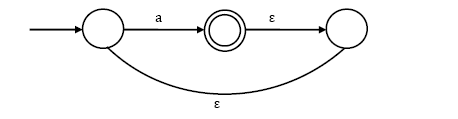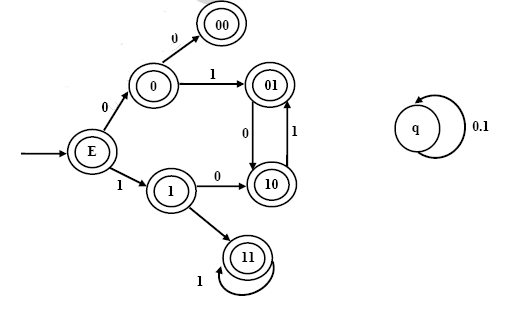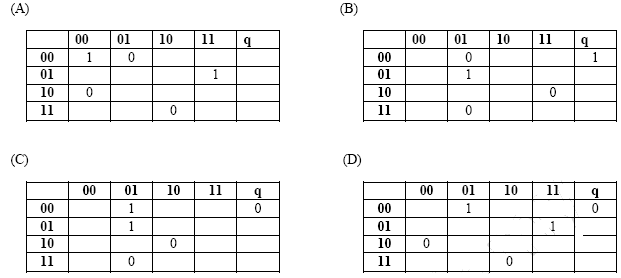# Automata Theory | Set 2

• Difficulty Level : Medium
• Last Updated : 21 Jul, 2021

Following questions have been asked in GATE CS 2012 exam.

1) What is the complement of the language accepted by the NFA shown below? Assume ∑ = {a} and ε is the empty string(A) Φ
(B) ε
(C) a
(D) {a, ε}

The given alphabet ∑ contains only one symbol {a} and the given NFA accepts all strings with any number of occurrences of ‘a’. In other words, the NFA accepts a+. Therefore complement of the language accepted by automata is empty string.

2) Given the language L = {ab, aa, baa}, which of the following strings are in L*?
….1) abaabaaabaa
….2) aaaabaaaa
….3) baaaaabaaaab
….4) baaaaabaa
(A) 1, 2 and 3
(B) 2, 3 and 4
(C) 1, 2 and 4
(D) 1, 3 and 4

Any combination of strings in set {ab, aa, baa} will be in L*.
….1) “abaabaaabaa” can be partitioned as a combination of strings in set {ab, aa, baa}. The partitions are “ab aa baa ab aa”
….2) “aaaabaaaa” can be partitioned as a combination of strings in set {ab, aa, baa}. The partitions are “aa ab aa aa”
….3) “baaaaabaaaab” cannot be partitioned as a combination of strings in set {ab, aa, baa}
….4) “baaaaabaa” can be partitioned as a combination of strings in set {ab, aa, baa}. The partitions are “baa aa ab aa”

3) Which of the following problems are decidable?
….1) Does a given program ever produce an output?
….2) If L is a context-free language, then is L’ (complement of L) also context-free?
….3) If L is a regular language, then is L’ also regular?
….4) If L is a recursive language, then, is L’ also recursive?
(A) 1, 2, 3, 4
(B) 1, 2,
(C) 2, 3, 4
(D) 3, 4

….1) Is a variation of Turing Machine Halting problem and it is undecidable.
….2) Context Free Languages are not closed under intersection and complement. See this for details.
….3) Complement of Regular languages is also regular. Then a DFA that accepts the complement of L, i.e. ∑* – L, can be obtained by swapping its accepting states with its non-accepting states.
….4) Recursive Languages are closed under complement. See this for details.

4) Consider the set of strings on {0,1} in which, every substring of 3 symbols has at most two zeros. For example, 001110 and 011001 are in the language, but 100010 is not. All strings of length less than 3 are also in the language. A partially completed DFA that accepts this language is shown below.The missing arcs in the DFA are